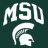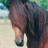# Murrey's Math Trend System for ThinkorSwim#### BenTen

Staff
VIP
This indicator was created & developed by Ucsgears, based on Murrey Math Line Principle.

Hello, Murrey Math lovers, Thanks for those who showed interest on this. Based on a request, I have updated the plot / candle coloring, for Version - 2. This has been in the queue for a while. There was a Glitch found with the Multiplier. Will Fix in the next version. The Current Version (and the previous version) only supports 1/8 fractions. Will not support 0.25. The code needs to be updated, to automate the fractal line glitches for other ratios, Planned for future update. Good Luck and Enjoy the Colorful Oscillator. Please keep your suggestions flowing. Lets make it better.Script #1: Simple. No lower study. Just paint bars (green = bullish and red = bearish)

Code:
``````# Murrey's Math Oscillator
# Assembled by BenTen at useThinkScript.com

# This is a stripped down or the original version. No lower study. Just simple paint bars.

input length = 100;
input mult = 0.125;

def hi = highest(high, length);
def lo = lowest(low, length);
def range = hi - lo;
def multiplier = (range) * mult;
def midline = lo + multiplier * 4;

def oscillator = (close - midline) / (range / 2);

def a = oscillator > 0 and oscillator<mult*2;
def b = oscillator > 0 and oscillator < mult*4;
def c = oscillator > 0 and oscillator < mult*6;
def d = oscillator > 0 and oscillator < mult*8;

def z = oscillator < 0 and oscillator > -mult*2;
def y = oscillator < 0 and oscillator > -mult*4;
def x = oscillator < 0 and oscillator > -mult*6;
def w = oscillator < 0 and oscillator > -mult*8;

assignPriceColor(if a or b or c or d then color.green else if w or z or y or x then color.red else color.white);``````

Script #2: Just paint bars. No lower study. But with color variations added.

Code:
``````# Murrey's Math Oscillator
# Assembled by BenTen at useThinkScript.com

# This is also a stripped down version. But it has color variations for paint bars.

input length = 100;
input mult = 0.125;

def hi = highest(high, length);
def lo = lowest(low, length);
def range = hi - lo;
def multiplier = (range) * mult;
def midline = lo + multiplier * 4;

def oscillator = (close - midline) / (range / 2);

def a = oscillator > 0 and oscillator<mult*2;
def b = oscillator > 0 and oscillator < mult*4;
def c = oscillator > 0 and oscillator<mult*6;
def d = oscillator > 0 and oscillator < mult*8;

def z = oscillator < 0 and oscillator > -mult*2;
def y = oscillator < 0 and oscillator > -mult*4;
def x = oscillator < 0 and oscillator > -mult*6;
def w = oscillator < 0 and oscillator > -mult*8;

assignPriceColor(if a then CreateColor(173, 255, 47) else if b then CreateColor(50, 205, 50) else if c then CreateColor(60, 179, 113) else if d then CreateColor(0, 128, 0) else if z then CreateColor(205, 92, 92) else if y then CreateColor(250, 128, 114) else if x then CreateColor(255, 160, 122) else if w then CreateColor(255, 0, 0) else color.white);``````

Script #3: Complete version. Just like the original script from TradingView.

Code:
``````# Murrey's Math Oscillator
# Assembled by BenTen at useThinkScript.com

declare lower;

input length = 100;
input mult = 0.125;

def hi = highest(high, length);
def lo = lowest(low, length);
def range = hi - lo;
def multiplier = (range) * mult;
def midline = lo + multiplier * 4;

def oscillator = (close - midline) / (range / 2);

def a = oscillator > 0 and oscillator<mult*2;
def b = oscillator > 0 and oscillator < mult*4;
def c = oscillator > 0 and oscillator<mult*6;
def d = oscillator > 0 and oscillator < mult*8;

def z = oscillator < 0 and oscillator > -mult*2;
def y = oscillator < 0 and oscillator > -mult*4;
def x = oscillator < 0 and oscillator > -mult*6;
def w = oscillator < 0 and oscillator > -mult*8;

plot histogram = oscillator;
histogram.SetPaintingStrategy(PaintingStrategy.HISTOGRAM);
histogram.AssignValueColor(if a then CreateColor(173, 255, 47) else if b then CreateColor(50, 205, 50) else if c then CreateColor(60, 179, 113) else if d then CreateColor(0, 128, 0) else if z then CreateColor(205, 92, 92) else if y then CreateColor(250, 128, 114) else if x then CreateColor(255, 160, 122) else if w then CreateColor(255, 0, 0) else color.white);

plot upper_l1 = mult*6;
plot upper_l2 = mult*8;
plot lower_l1 = -mult*6;
plot lower_l2 = -mult*8;

upper_l1.AssignValueColor(color.gray);
upper_l2.AssignValueColor(color.gray);
lower_l1.AssignValueColor(color.gray);
lower_l2.AssignValueColor(color.gray);

#assignPriceColor(if a or b or c or d then color.green else if w or z or y or x then color.red else color.white);

assignPriceColor(if a then CreateColor(173, 255, 47) else if b then CreateColor(50, 205, 50) else if c then CreateColor(60, 179, 113) else if d then CreateColor(0, 128, 0) else if z then CreateColor(205, 92, 92) else if y then CreateColor(250, 128, 114) else if x then CreateColor(255, 160, 122) else if w then CreateColor(255, 0, 0) else color.white);``````#### J007RMC

##### Well-known member
2019 Donor
VIP
Ive read on Murry's lines where each line is proportional to 1/8. from what I can tell
Oscillator Color Definition -
Green = Above MidLine
Red = Below Midline
Blue = Below Negative 3rd Quadrant
Orange = Above Positive 3rd Quadrant

Last edited:
•markos
D

#### diazlaz

##### Well-known member
2019 Donor
VIP
based on research and some testing, I find in the lower time frame, it does a pretty good job finding tops and bottoms on OB and OS conditions.

if oscillator crosses below (mult * 6) then short else
if oscillator crosses above (-mult * 6) then long.

if anyone has any other test results or experiences please share.

•J

#### john3

##### Active member
2019 Donor
Apart from circles, speed lines, and frames, Murrey math is the same as Fibonacci ratios.

L

#### Lambert58

##### New member
Do we have the original Murrey math with all of the levels for TOS, the video's for the original are extensive and detailed. The levels make the application clearer for me.

Thanks#### BenTen

Staff
VIP
@Lambert58 Not in this one.

L

#### Lambert58

##### New member
John3, this video link for Murrey Math is a detailed explanation, a little long.

Let me know if this is useful for you.

•Beltrame1 and J007RMC
J

#### john3

##### Active member
2019 Donor
@Lambert58 Thank you for the video. I've seen it before. I thought maybe you were talking about a video that I haven't seen.

That and all other videos/discussions of Murrey Math/Fibs miss several key points, i.e., they don't discuss progression and how the price interacts with key injection pivots.

T

#### TK_44

##### New member
VIP
@Lambert58 Are you pretty familiar with murrey math? I have been checking into this and can't seem to figure out how the lines are technically supposed to be drawn. What defines the 0/8 and 8/8 range? Where do they start from, or in other words what defines the high-low range? From what I can tell it can't be a simple high-low range of a look back period of 100 like in this indicator because it doesn't allow +1/8, +2/8, -1/8, -2/8, etc. levels.

H

#### henry1224

##### Member
VIP
While scripting this code into Edgerater & TOS I have noticed that this Oscillator resembles the outcomes of a slow stochastics (10,3,3) & (50,3,3)••Beltrame1 and BenTen
W

#### WayneG

##### New member
VIP
Looks really good. Do you have links to the Murry Math and HK Slow Stochastic? I would really appreciate it.

wayneG

H

#### henry1224

##### Member
VIP
I used the same code as above. Instead of coloring the Histogram bars, I used a moving average of the histogram and added the cloud feature,
But the signals are very similar to a slow stochastic. I used 2 slow stochastics (10,3,,3) & (50,3,3) and I also use the cloud feature for those. a darker cloud for the longer time length

W

#### WayneG

##### New member
VIP
I used the same code as above. Instead of coloring the Histogram bars, I used a moving average of the histogram and added the cloud feature,
But the signals are very similar to a slow stochastic. I used 2 slow stochastics (10,3,,3) & (50,3,3) and I also use the cloud feature for those. a darker cloud for the longer time length
Sorry, but these numbers, (10,3,,3) & (50,3,3), don't give the results shown in the images. wayneG

H

#### henry1224

##### Member
VIP
Hello Wayne

Here is the Edgerater Script for the Murrey Math Osc:

Code:
``````hi:=HHV(High,length);
lo:=LLV(Low,length);
range:= hi - lo;
multiplier:= (range) * mult;
midline:= lo + multiplier * 4;
MMO : close - midline) / (range / 2),width2,ColorBlue;
MMOA: MA(MMO,Length2),Width2,ColorRed;
MX:=Max(MMO,MMOA);
MN:=Min(MMO,MMOA);
uZ1 : mult*6,Width2,ColorBlack;
uZ2 : mult*8,Width2,ColorBlack;
lZ1 : -mult*6,Width2,ColorBlack;
lZ2 : -mult*8,Width2,ColorBlack;
FillRgn(1,UZ1,UZ2),Brush#50FFA500;
FillRgn(1,LZ1,LZ2),Brush#50FFA500;
FillRgn(MMO>MMOA,MMO,MMOA),Brush#800000FF;
FillRgn(MMO<MMOA,MMO,MMOA),Brush#80FF0000;
FillRgn(MN>0,MN,0),Brush#200000FF;
FillRgn(MX<0,MX,0),Brush#20FF0000;``````

And here is the Edgerater code for HK Slow Stochastics:

Code:
``````K:=(Close-LLV(Low,LB))/(HHV(H,LB)-LLV(Low,LB))*100,Width2,ColorBlue;
D:Ma(K,N1),Width2,ColorGreen;
DS:MA(D,N2),Width2,ColorBlack;

WK:=(Close-LLV(Low,WLB))/(HHV(H,WLB)-LLV(Low,WLB))*100,Width2,ColorBlue;
WD:Ma(WK,N1),Width2,ColorBlue;
WDS:MA(WD,N2),Width2,ColorRed;

EightyLine:80,Width2,ColorRed;
FiftyLine:50,ColorBlack;
twentyLine:20,Width2,ColorGreen;

@SETHLINE(20,50,80);
FillRgn(D>DS,D,DS),Brush#300000FF;
FillRgn(D<DS,D,DS),Brush#30FF0000;

FillRgn(Min(D,DS)>80,Min(D,DS),80),Brush#50FFA500;
FillRgn(Max(D,DS)<20,Max(D,DS),20),Brush#7000FF00;

FillRgn(WD>WDS,WD,WDS),Brush#800000FF;
FillRgn(WD<WDS,WD,WDS),Brush#80FF0000;

FillRgn(Min(WD,WDS)>80,Min(WD,WDS),80),Brush#50FFA500;
FillRgn(Max(WD,WDS)<20,MAX(WD,WDS),20),Brush#7000FF00;``````

I cannot vouch for how TOS plots these indicators? But using Edgerater, the indicators line up very close to each other! just saying.

W

VIP
Thanks

S

#### SparkyFlary

##### Member
I've noticed in other codes that whenever Highest(price,length) and Lowest(price,length) are used, they're usually done like Highest(price,length) and Lowest(price,length). Will keeping it as is without the brackets at the end cause it to repaint? Just want to make sure it doesn't repaint.RTH High Low (Murrey Math / Donchian ) Strategy Strategies 1Murrey Math Harmonics for ThinkorSwim Indicators 19